Volume 63 Supplement 1

# Lunar ionosphere exploration method using auroral kilometric radiation

## Abstract

The evidence of a lunar ionosphere provided by radio occultation experiments performed by the Soviet spacecraft Luna 19 and 22 has been controversial for the past three decades because the observed large density is difficult to explain theoretically without magnetic shielding from the solar wind. The KAGUYA mission provided an opportunity to investigate the lunar ionosphere with another method. The natural plasma wave receiver (NPW) and waveform capture (WFC) instruments, which are subsystems of the lunar radar sounder (LRS) on board the lunar orbiter KAGUYA, frequently observe auroral kilometric radiation (AKR) propagating from the Earth. The dynamic spectra of the AKR sometimes exhibit a clear interference pattern that is caused by phase differences between direct waves and waves reflected on a lunar surface or a lunar ionosphere if it exists. It was hypothesized that the electron density profiles above the lunar surface could be evaluated by comparing the observed interference pattern with the theoretical interference patterns constructed from the profiles with ray tracing. This method provides a new approach to examining the lunar ionosphere that does not involve the conventional radio occultation technique.

## 1. Introduction

The lunar atmosphere is extremely tenuous compared to the Earth’s atmosphere. The gas concentration, which consists of helium, argon, etc. (Stern, 1999), is only approximately 2 × 105 cm−3 during the lunar night and 104 cm−3 during the lunar day (Hodges, 1975). Like the Earth’s ionosphere, these gas particles are ionized by solar ultraviolet radiation to form the ionosphere. In theory, however, the peak density in the lunar ionosphere is considered to be less than or equal to the solar wind density. Nevertheless, it was reported that 500 to 1000 cm−3 electron densities were observed at altitudes of 5 to 10 km by the Soviet Luna 19 and 22 in the 1970s (Vasil’ev et al., 1974; Vyshlov and Savich, 1979). In this observation, an occultation technique was used to measure the phase shifts of radio waves due to electron densities around the Moon. This result remains controversial, however, because the observed large density is difficult to explain theoretically without magnetic shielding from the solar wind.

Because the Moon does not have a strong global magnetic field like the Earth, the interplanetary magnetic field (IMF) principally determines the magnetic field around the Moon. In local field structures, however, there are some regions that have strong magnetic fields, called mini-magnetospheres (Hood and Schubert, 1980; Hood et al., 2001; Kurata et al., 2005). The dense plasma layers may be maintained by these strong fields. Alternatively, near-surface electric fields could possibly loft charged particles up to higher altitudes (Stubbs et al., 2006). The high densities observed by the Luna are therefore a result of a currently unknown maintainable or transfer mechanism of charged particles.

During the KAGUYA mission, as in the Luna experiments, an electron density profile above the lunar surface was observed by a radio occultation technique using a Vstar sub-satellite (Imamura et al., 2008). In this experiment, coherent S-band and X-band signals were employed to measure the total electron content from the satellite to a terrestrial tracking station. Because the density of the lunar ionosphere can be obtained by subtracting the effects of the Earth’s ionosphere, the estimation accuracy of this technique depends on that of the Earth’s ionosphere.

The KAGUYA continuously observed natural waves using the natural plasma wave receiver (NPW) and the waveform capture (WFC) instruments, which are subsystems of the lunar radar sounder (LRS) (Kasahara et al., 2008; Ono et al., 2008, 2010). Auroral kilometric radiation (AKR), whose source is the Earth’s polar regions, is one of these waves. The AKR frequency is considered to correspond to the local electron gyro frequency at the source on the auroral field lines (Morioka et al., 2005). The source of the AKR in a frequency range from 100 to 500 kHz is, therefore, located at an altitude range from 3,500 to 10,000 km. As depicted in the time-frequency spectrogram in Fig. 1, the AKR observed by the KAGUYA sometimes includes a clear interference pattern. This pattern is caused by phase differences between directly arrived waves and waves reflected on or near the lunar surface, as illustrated in Fig. 2. Actually, the peak electron densities observed by the Luna correspond to plasma frequencies from 200 to 450 kHz. Reflection always occurs on such density layers when the AKR frequency is lower than these plasma frequencies. However, even when the AKR frequency is higher than the plasma frequencies, reflection occurs in the case of oblique incidences. Therefore, the interference patterns signify the existence of the lunar ionosphere in certain conditions.

In this paper, a new exploration method of the lunar ionosphere using the AKR is presented. A theoretical interference pattern of the AKR for a given electron density profile can be constructed by calculating the phase delays of reflected waves to direct waves with ray tracing. Then, possible density profiles can be evaluated by comparing the observed interference patterns with the theoretical ones reconstructed for the profiles. This is a new approach to examining the lunar ionosphere that is not based on the radio occultation technique.

## 2. Instrumentation and Data

The KAGUYA is a lunar orbiter that is placed in a polar orbit with an inclination of 90° and a nominal altitude of 100 km from the lunar surface. The orbit covers all local times in half a month. The NPW and WFC, both of which receive natural plasma waves, are subsystems of LRS on board the KAGUYA. Both receivers obtain electric field spectrograms and waveforms with two orthogonal 30 m tip-to-tip antennas. The NPW covers a frequency range from 20 kHz to 10 MHz, and the WFC covers that from 100 Hz to 1 MHz. Various kinds of observation modes are available in both receivers. Although waveform observation by the NPW is the best at analyzing the interference pattern of the AKR in terms of time and frequency resolutions, data coverage is unfortunately not very extensive. In contrast, although the resolutions of the WFC spectrogram are poor, the amount of data is sufficient to enable statistical study.

From the upper hybrid resonance frequency in the spectrogram of the WFC, in situ electron density is estimated along a satellite trajectory. The observed density exhibits a clear day-night asymmetry. The day-side density is generally Ne = 1–5 cm−3 whereas the night-side density is Ne < 0.1 cm−3. The day-side density is considered to denote the solar wind density and the night-side density is consistent with the lunar wake.

Magnetic field strength at the KAGUYA trajectory is governed by the IMF when the KAGUYA is not located within the Earth’s magnetosphere. The strength is usually less than 10 nT, and thus, the electron gyro frequency, which is calculated as frequency less than 280 Hz, is sufficiently small relative to the AKR frequency. Therefore, the plasma environment around the Moon is considered to be isotropic for the AKR. The refractive index of the AKR wave can be regarded as a function of only in situ electron plasma frequency.

## 3. Theoretical Calculation of Phase Difference

A principal problem in exploring the lunar ionosphere using the AKR is calculating theoretical phase patterns accurately. For this purpose, ray tracing was used. In this section, after describing the ray tracing, phase maps are uniquely constructed for typical electron density profiles observed by the Luna.

### 3.1 Ray tracing

The interference pattern of the AKR is caused by phase differences between direct and reflected waves. These phase differences are determined by path differences between these two waves and the reflectance of the lunar surface. If the AKR propagates in a mode that approximates light speed around the Moon, the path differences can be geometrically calculated. However, if the dispersion of plasma must be taken into account, ray tracing is convenient for calculating the path difference accurately. Because the purpose of ray tracing here is to estimate the propagation delay of the reflected waves to the direct waves, their propagations near the Earth are not dealt with strictly. These waves propagate along almost the same trajectory there. Therefore, the ray tracing is started from where the AKR begins to propagate along a straight path. This means that the starting position of the ray tracing is not a real wave source but a virtual one. The validity of the virtual source is discussed in 3.4.

In Fig. 3, a two-dimensional orthogonal coordinate system X Z is defined as a ray tracing space, where the center of the Moon is at the coordinate origin O, a virtual wave source S is on the X axis and the KAGUYA location P is on the X Z plane. An angle η ≡ POS to denote the position of the KAGUYA is also defined. In the numerical calculation of the ray tracing, the orthogonal coordinate system (X, Z) is converted into a polar coordinate system (r, ϕ) by functions of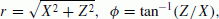(1)

for convenience. Then, the position of a traced ray is represented by the distance from the origin r and the angle from the X axis ϕ, as depicted in Fig. 3. The wave normal angle of the ray is defined by the angle δ between the wave normal vector and the position vector (r, ϕ).

Propagation paths are calculated recursively from an initial position (r0, ϕ0) and an initial wave normal angle δ0 using the following differential equations (Kimura et al., 1985):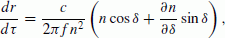(2)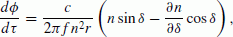(3)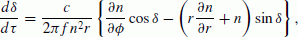(4)

where τ is a non-dimensional parameter that is proportional to a distance along the propagation path. f is a wave frequency and η is a refractive index, which is represented by the following equation in isotropic plasma (Stix, 1962):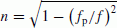(5)

where fp is in situ plasma frequency. In this study, a modified Adams’ method was adopted to solve these differential equations (Shampine and Gordon, 1975).

### 3.2 Time delay of reflected AKR for density profiles observed by the luna mission

In order to examine the feasibility of the new approach, three typical vertical density profiles (Profile 0, 1, 2) from the Luna observations and one vertically uniform profile of 5cm−3 (Profile 3), as illustrated in Fig. 4, were assumed. Profile 3 assumes the background solar wind electron density. The former three profiles have peak densities of 2,200 cm−3, 750 cm−3, and 450 cm−3 at an altitude of 5 km, respectively. In each model, density falls into the solar wind density of 5 cm−3 smoothly at high altitudes. For simplicity, all profiles are assumed to be spherically symmetric. The AKR is reflected on a layer whose plasma frequency corresponds to wave frequency when a sufficiently dense ionosphere exists. In contrast, the AKR is reflected on the lunar surface when the lunar ionosphere does not exist or when it is too tenuous. In this case, attenuation and a phase shift due to the reflection occur to the wave. In this study, relative permittivity and conductivity on the lunar surface was assumed to be ɛ = 10 and σ = 10−13 m−1, respectively (Carrier et al., 1991).

Ray tracing was performed from an initial position represented as S in Fig. 3. The initial distance r0 from the center of the Moon was set to be the distance between the Earth and the Moon at the period of every AKR observation. The distance was not so sensitive to time delays of the reflected waves to direct waves. The altitude of the KAGUYA was assumed to be 100 km here. Because the altitude actually varies within 100 ± 20 km, it is necessary to recalculate the theoretical delays for a real altitude in application. The propagation times of the direct and reflected waves to the observation location P were calculated by changing the initial wave normal angle δ0 until the ray path intercepted the location P.

Figures 5, 6, and 7 depict the time delays for satellite position η for wave frequencies of 100 kHz, 200 kHz, and 400 kHz, respectively. In the figures, there is no difference between time delays for 100 kHz, 200 kHz and 400 kHz for Profile 3, as represented by solid lines. This is due to the uniform density profile and frequency-independent reflection on the lunar surface because of a small conductivity. The delay is long for small η. The longest delay is found when the AKR comes from the zenith direction (η = 0°). This tendency can be easily understood because the difference in path lengths between direct and reflected waves becomes small as η becomes larger. The direct and reflected waves cannot be distinguished around η = 110° because the observation point is concealed behind the Moon.

From the result of f = 100 kHz, the delay becomes shorter in the following order: Profile 1, 0, 2, 3. The plasma frequency fp is 100 kHz at altitudes of 34 km, 27 km, and 23 km for Profile 1, 0, and 2, respectively. Thus, the order is considered to be determined by the reflection altitude. When the AKR comes from the zenith direction for Profile 1, the delay is 0.5 ms. This takes 10% or more additional time compared to the case in which the wave propagates to the reflection altitude at the light speed because the group refractive indices above the reflection point are larger than unity. The same tendency can be observed for Profile 0 and 2.

Next, from the result of f = 200 kHz, the delay for each profile is in the same order as that of 100 kHz for 13° <η whereas the delays for Profile 0 and 1 are counterchanged for η < 13°. Although the plasma frequency is 200 kHz at 20 km in both Profile 0 and 1, the delay for Profile 1 is longer than that for Profile 0 for η 0°. This is because the group refractive index for Profile 1 is larger than that for Profile 0 above 20 km. For oblique incidence, however, the reflection altitude for Profile 1 is higher than that for Profile 0, in accordance with Snell’s law. Thus, the delay for Profile 0 is longer than Profile 1 for large η. Because the peak plasma frequency for Profile 2 is not higher than 200 kHz, the wave is considered to reach the lunar surface for η 0°. The delay for Profile 2, however, does not agree with that for Profile 3, even when η = 0°.

Finally, from the result of f = 400 kHz, all delay curves for Profile 0, 1, and 2 approach that for Profile 3. Because fp/f is relatively small compared to that for f = 100 kHz and 200 kHz, the refractive index of the wave approaches unity and the group velocity approaches light speed. For η 0°, the ionospheric reflection occurs only in Profile 0 and the surface reflection occurs in Profile 1, 2, and 3. It should be noted that, in the case of the surface reflection, the delay for Profile 1 and 2 never agrees with that for Profile 3.

On the basis of these results, it is concluded that the delay curve for η signifies both reflection altitude and vertical density profiles. The delay is different even if the reflection altitude is the same because the electron density above the altitude influences the wave propagation. In addition, for oblique incidence, even when the peak of the electron density profile is not very large, ionospheric reflection occurs.

### 3.3 Construction of the phase difference map

The presence of the interference pattern observed by the KAGUYA implies that there is a standing wave between the lunar surface and the observation altitude. This pattern is not determined by an initial phase at a wave source but by an electron density profile above the lunar surface and its reflectivity.

The phase difference between the direct and reflected waves can be calculated by multiplying the delay to the corresponding angular frequency. The phase shift due to reflectance of the lunar surface is added if necessary. Based on these calculations, “phase maps” for wave frequency f and satellite position η can be obtained, as shown in Fig. 8. Each map represents the phase difference for Profile 0, 1, 2, and 3 by tone in a range of 90° < η < 100° and 100 kHz < f < 500 kHz. In the maps, the black and white parts indicate that the phase difference equals 0 and π, respectively. The reason why only a range of 90° < η < 100° is shown is that it is easy to observe a clear interference pattern in this frequency range.

In the phase maps, there is a difference in stripe intervals between the cases in which the lunar ionosphere exists (Profile 0, 1, 2) and the cases in which it does not exist (Profile 3). When the KAGUYA moves from η = 90° to 100°, for example, the number of stripe changes is as shown in Table 1. Each number variation for η is considered to reflect the density profile below the observation point. Because the delay for Profile 3 is the longest among the given profiles except for η 0° and high frequencies from Figs. 5 to 7, the number for Profile 3 is almost always the greatest. The frequency intervals of the stripes in the phase maps generally become the minimum at η = 0° and become larger as η becomes larger.

As a result, if η is obtained at an observation point, a reasonable density profile can be chosen from the interference pattern with this phase-map-fit method. Because η is an angle in the coordinate system defined by using a wave source, unfortunately, an approximate value of it has to be used instead.

### 3.4 Source location

Figure 9 presents a three-dimensional coordinate system xyz where the center of the Moon is the coordinate origin, the center of the Earth (E) is on the x axis, and an initial position of ray tracing (S′) is on the xz plane. As previously described, S′ does not indicate a real source of the AKR. The observation point is defined as P′. Whereas the two-dimensional plane of the ray tracing is redefined by O, P′, and S′, the angle η = S′OP′ cannot be obtained by the orbit data of the KAGUYA. Only an angle η′ = EOP′ is obtained by the orbit data. Considering that the distance between the Earth and the initial position of ray tracing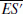is much shorter than that between the Earth and the Moon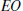, a difference of Δηη′η is not very large.

Δη is a function of an observation point P′(p x , p y , p z ) and an initial position of ray tracing S′(s x , 0, s z ). If the altitude of the KAGUYA is assumed to be 100 km here, two parameters η′ and ψ can be used instead of p x , p y , and p z . This assumption does not make a critical difference in the following discussion. ψ indicates the rotation angle of the KAGUYA to the x axis, as depicted in Fig. 9. ψ = 0° and 90° indicate that the KAGUYA is located on the xy plane and xz plane, respectively. Figure 10 presents the Δη maps for the parameters η′, ψ, s x , and s z . The three rows correspond to η′ = 30°, 60°, and 90°. The four columns correspond to ψ = 0°,30°,60° and 90°. In each map, Δη is expressed by tones at the initial position of ray tracing (sx, sz). A semicircle on the left side of each map denotes the Earth. The horizontal and vertical lines are parallel to the x and z axes in the coordinate system in Fig. 9, respectively.

Comparing upper, middle, and lower four maps, Δη is found to be almost the same in each column. This implies that the variation of η′ does not have to be taken into consideration. The figure also reveals that the variation of Δη for s x is small. This is owing to a contribution of s x to Δη. Δη is actually a function of s z /s x in which s x is nearly 60 Earth radius. Δη is approximately estimated by the two parameters s z and ψ.

In previous ray tracing studies of the AKR, waves were strongly refracted in the vicinity of the source (Hashimoto, 1984). After they propagate 1 to 2 Earth radius away from the Earth, the refraction becomes small and the waves propagate along straight lines. Thus, the virtual source S′ should be put on a position at a distance within 3.5 Earth radius from the x axis. Under the condition, |Δη| < 1° for ψ = 0°, |Δη| < 2° for ψ = 30°, |Δη| < 3° for ψ = 60°, and |Δη| < 4° for ψ = 90°. The sign of Δη, which is positive when p z and s z are the same sign and negative when p z and s z are the opposite sign, can be provided by an occultation observation of the AKR. It should be noted that these error ranges include the frequency dependence of the wave source.

Δη is a dominant error factor in the construction of a theoretical phase pattern. The error range can be taken into account by making a theoretical phase map that is calculated under the assumption of the Δη = 0° shift along the η axis within Δη. Actually, even if Δη > 3°, there is a case in which one can determine whether or not the ionosphere exists. It is, however, difficult to distinguish similar electron density profiles for large Δη.

## 4. Example Application

The proposed method was applied to the observation data depicted in Fig. 1. During this period, the KAGUYA was orbiting in a midnight region at altitudes of 87 to 88 km and η′ is varied from 100.7° at 12:58 UT to 97.1° at 13:01 UT. By assuming Δη = 0°, theoretical phase patterns were constructed during this period for Profile 0, 1, 2, and 3, as shown in Fig. 11. Because ψ is supposed to be in 0° < ψ < 30° from the KAGUYA orbit, |Δη| is considered to be smaller than 2°. That is, the phase pattern can be shifted along a time sequence within approximately 2 min.

In each figure in Fig. 11, the observed pattern and theoretical phase patterns are represented by black lines and tones, respectively. The number of stripes exhibits good correspondence between the observed pattern and theoretical ones for Profile 3. Even if the theoretical phase patterns for Profile 0, 1, and 2 are shifted by ±2 min, the number of stripes does not agree with that of the observed one. Therefore, it is concluded that a dense ionosphere like that in the Luna observation did not exist in this region. Because the high electron densities measured by the Luna missions were only observed above the sunlit lunar surface and the KAGUYA was orbiting in the midnight region when the example spectrogram was observed, this result is consistent with past various radio occultation observations.

## 5. Discussion and Conclusions

In this paper, a new lunar ionosphere exploration method using the AKR propagating from the Earth was proposed. The method is based on a kind of model fitting in which candidate density profiles are evaluated by the correspondence between an observed interference pattern and theoretically calculated ones from the profiles. Using the AKR spectrogram observed by the KAGUYA, the practical use of the method was demonstrated. In the future, we plan to examine the existence of the ionosphere using more AKR data obtained by the KAGUYA and to conduct a statistical study. The KAGUYA actually had an opportunity to observe electron densities at low altitudes at the end of the mission although the surveyed regions were limited. According to the first analysis, no evidence of a high electron density layer near the lunar surface like that in the Luna measurements has been found.

In the case where the frequency resolution of the observed spectrogram is not very good, it is effective to deal with the observation data for 80° < η. Under this condition, an interference pattern in which the frequency interval is from 10 to 100 kHz is easily found. The condition is satisfied twice per orbit. The time duration depends on the angle between the orbital plane of the KAGUYA and the x axis in Fig. 9. When the angle is near 90°, the condition is always satisfied. Because the condition is independent of the direction of the sun, both regions at day-side and along the boundary between day-side and night-side can be covered with such helpful data.

Another advantage of using the observation data for large η is that the influence of the altitude error of the KAGUYA is reduced. In the example application, the Moon was assumed to be a sphere and the altitude was estimated from the orbit data and the average radius of the Moon. More accurate calculation of the delay of the reflected wave can be realized by utilizing the real shape of the Moon. For this purpose, it is effective to use the observation data of the laser altimetry (LALT) equipment on board the KAGUYA.

The recent study that estimated the AKR source through occultation observation from the KAGUYA has been useful in improving the accuracy of the proposed method because the AKR source is a dominant error factor. The distribution of the arrival angle of the AKR and the distribution of frequencies also improve the accuracy of the method.

## References

• Carrier III, W. D., G. R. Olhoeft, and W. Mendell, Physical properties of the lunar surface, in Lunar Source Book, edited by G. H. Heiken, D. T. Vaniman, and B. M. French, 736 pp, Cambridge University Press, USA, 1991.

• Hashimoto, K., A reconciliation of propagation modes of auroral kilometric radiation, J. Geophys. Res., 89 (A9), 7459–7466, 1984.

• Hodges, R. R. Jr., Formation of the lunar atmosphere, The Moon, 14, 139–157, 1975.

• Hood, L. L. and G. Schubert, Lunar magnetic anomalies and surface optical properties, Science, 208, 49–51, 1980.

• Hood, L. L., A. Zakharian, J. Halekas, D. L. Mitchell, R. P. Lin, M. H. Acuna, and A. B. Binder, Initial mapping and interpretation of lunar crustal magnetic anomalies using Lunar Prospector magnetometer data, J. Geophys. Res., 106, 27825–27839, 2001.

• Imamura, T., K.-I. Oyama, T. Iwata, Y. Kono, K. Matsumoto, Q. Liu, H. Noda, Y. Futaana, and A. Nabatov, The possibility of studying the lunar ionosphere with the SELENE radio science experiment, Earth Planets Space, 60, 387–390, 2008.

• Kasahara, Y., Y. Goto, K. Hashimoto, T. Imachi, A. Kumamoto, T. Ono, and H. Matsumoto, Plasma wave observation using waveform capture in the Lunar Radar Sounder on board the SELENE spacecraft, Earth Planets Space, 60, 341–351, 2008.

• Kimura, I., T. Matsuo, M. Tsuda, and K. Yamauchi, Three dimensional ray tracing of whistler mode waves in a non-dipolar magnetosphere, J. Geomag. Geoelectr., 37, 945–956, 1985.

• Kurata, M., H. Tsunakawa, Y. Saito, H. Shibuya, M. Matsushima, and H. Shimizu, Mini-magnetosphere over the Reiner Gamma magnetic anomaly region on the Moon, Geophys. Res. Lett., 32, L24205, doi:10.1029/2005GL0244097, 2005.

• Morioka, A., Y. S. Miyoshi, F. Tsuchiya, H. Misawa, A. Kumamoto, H. Oya, H. Matsumoto, K. Hashimoto, and T. Mukai, Auroral kilometric radiation activity during magnetically quiet periods, J. Geophys. Res., 110, A11223, doi:10.1029/2005JA011204, 2005.

• Ono, T., A. Kumamoto, Y. Yamaguchi, A. Yamaji, T. Kobayashi, Y. Kasahara, and H. Oya, Instrumentation and observation target of the Lunar Radar Sounder (LRS) experiment on-board the SELENE spacecraft, Earth Planets Space, 60, 321–332, 2008.

• Ono, T., A. Kumamoto, Y. Kasahara, Y. Yamaguchi, A. Yamaji, T. Kobayashi, S. Oshigami, H. Nakagawa, Y. Goto, K. Hashimoto, Y. Omura, T. Imachi, H. Matsumoto, and H. Oya, The Lunar Radar Sounder (LRS) onboard the KAGUYA (SELENE) spacecraft, Space Sci. Rev., doi:10.1007/s11214-010-9673-8, 2010.

• Shampine, L. F. and M. K. Gordon, Computer Solution of Ordinary Differential Equations, 318 pp, W. H. Freeman & Co. Ltd., 1975.

• Stern, S. A., The lunar atmosphere: history, status, current problems, and context, Rev. Geophys., 34 (4), 453–491, 1999.

• Stix, T. H., The Theory of Plasma Waves, 283 pp, McGraw-Hill Book Company, New York, 1962.

• Stubbs, T. J., R. R. Vondrak, and W. M. Farrell, A dynamic fountain model for lunar dust, Adv. Space Res., 37,59–66, 2006.

• Vasil’ev, M. B., V. A. Vinogradov, A. S. Vyshlov, O. G. Ivanovskii, M. A. Kolosov, N. A. Savich, V. A. Samovol, L. N. Samoznaev, A. I. Sidorenko, A. I. Sheikhet, and D. Ya. Shtern, Radio transparency of circumlunar space using the Luna-19 station, Cosmic Res., 12, 102–107, 1974.

• Vyshlov, A. S. and N. A. Savich, Observations of radio source occultations by the moon and the nature of the plasma near the moon, Cosmic Res., 16, 450–454, 1979.

## Acknowledgments

The authors would like to express their appreciation to Professor Kozo Hashimoto for his valuable comments. The KAGUYA (SELENE) project is organized by the Japan Aerospace Exploration Agency (JAXA). The authors would like to express their thanks to all members of the KAGUYA (SELENE) project team.

## Author information

Authors

### Corresponding author

Correspondence to Yoshitaka Goto.

## Rights and permissions

The images or other third party material in this article are included in the article’s Creative Commons licence, unless indicated otherwise in a credit line to the material. If material is not included in the article’s Creative Commons licence and your intended use is not permitted by statutory regulation or exceeds the permitted use, you will need to obtain permission directly from the copyright holder.

To view a copy of this licence, visit https://creativecommons.org/licenses/by/4.0/.

Reprints and Permissions

Goto, Y., Fujimoto, T., Kasahara, Y. et al. Lunar ionosphere exploration method using auroral kilometric radiation. Earth Planet Sp 63, 47–56 (2011). https://doi.org/10.5047/eps.2011.01.005

• Revised:

• Accepted:

• Published:

• Issue Date:

• DOI: https://doi.org/10.5047/eps.2011.01.005

### Key words

• KAGUYA
• lunar ionosphere